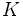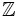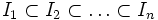Difference between revisions of "Dedekind domain"

This article defines a property of integral domains, viz., a property that, given any integral domain, is either true or false for that.
View other properties of integral domains | View all properties of commutative unital rings
VIEW RELATED: Commutative unital ring property implications | Commutative unital ring property non-implications |Commutative unital ring metaproperty satisfactions | Commutative unital ring metaproperty dissatisfactions | Commutative unital ring property satisfactions | Commutative unital ring property dissatisfactions

Definition

Symbol-free definition

An integral domain is termed a Dedekind domain if it satisfies the following equivalent conditions:

Relation with other properties

Stronger properties

Property Meaning Proof of implication Proof of strictness (reverse implication failure) Intermediate notions
Euclidean domain integral domain that admits a Euclidean norm click here
principal ideal domain integral domain in which every ideal is a principal ideal Dedekind not implies PID click here
polynomial ring over a field ring of the form$K[x]$ where$K$ is a field click here
ring of integers in a number field it is the integral closure of$\mathbb{Z}$ inside a number field (a finite degree field extension of the rationals) click here

Weaker properties

Property Meaning Proof of implication Proof of strictness (reverse implication failure) Intermediate notions
normal ring integrally in its total quotient ring click here
normal domain integrally closed in its field of fractions click here
Noetherian ring every ideal is finitely generated click here
one-dimensional ring the Krull dimension is at most one, i.e., we cannot have a strictly ascending chain$P_0 \subset P_1 \subset P_2$ of prime ideals click here
Noetherian domain integral domain and a Noetherian ring click here
one-dimensional domain integral domain and a one-dimensional ring. Explicitly the prime ideals are precisely the zero ideal and nonzero maximal ideals. click here
one-dimensional Noetherian domain integral domain that is a Noetherian ring and a one-dimensional ring. click here
Noetherian normal domain integral domain that is a Noetherian ring and a normal ring. click here

Conjunction with other properties

Any unique factorization domain which is also a Dedekind domain, is also a principal ideal domain.

Module theory

Any finitely generated module$M$ over a Dedekind domain$R$ can be expressed as a direct sum as follows:$M \cong R/I_1 \oplus R/I_2 \oplus \ldots \oplus R/I_n$

where$I_1 \subset I_2 \subset \ldots \subset I_n$ is an ascending chain of ideals, which could reach$R$.